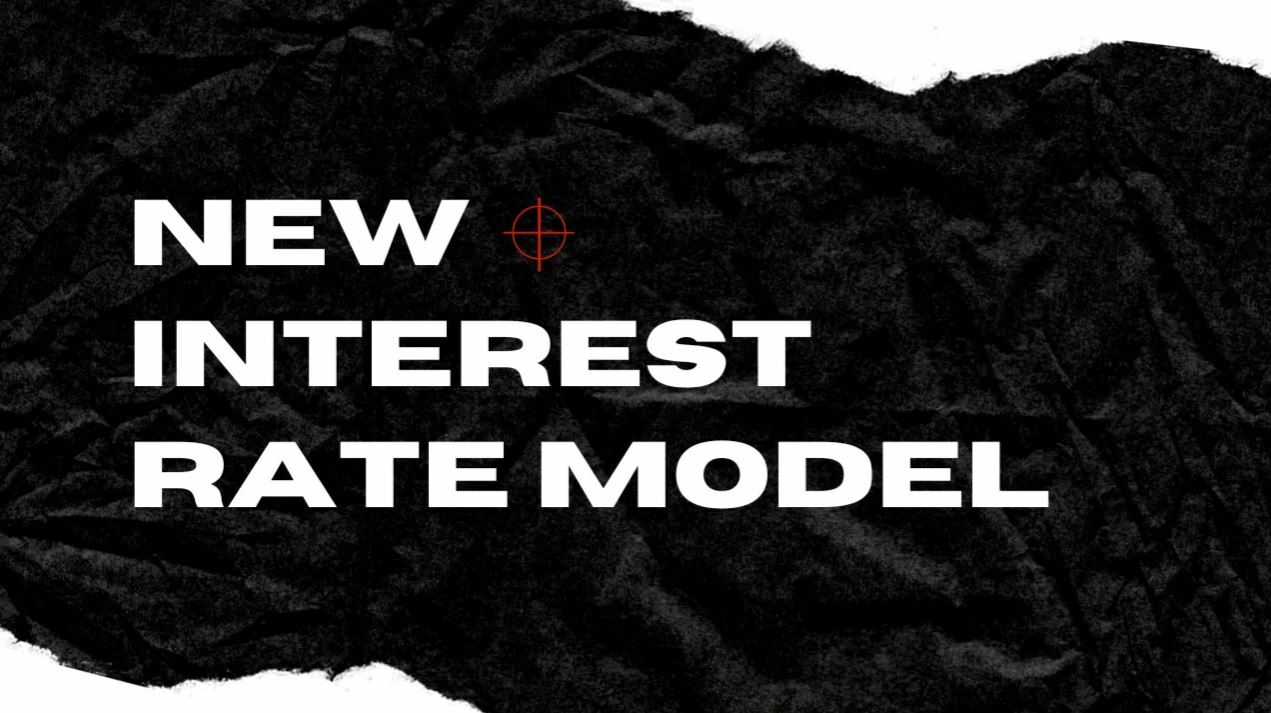Fringe Finance DeFi Blog

# Announcing Fringe’s improved interest rate model### Snapshot of our current situation

Until now, Fringe’s PLP has used Compound’s interest rate model, which can be optimized to significantly improve capital efficiency. As a result, we’ve developed an improved interest rate model, which is in testing mode and will be deployed to Mainnet SOON™.
Before proceeding, it is important to clarify what is meant when we use the term “utilization rate”. Utilization rate refers to the percentage of lender funds that are currently being borrowed. E.g. if lenders have deposited \$100, and \$20 has been loaned out, the utilization rate would be 20%.

### Why utilization rate?

Utilization rate, as a figure, is important as it signifies how efficiently lender funds are being used. If only 10% of lender funds are being loaned out (implying a utilization rate of 10%), 90% are sitting idle, and not earning any yield. The purpose of Fringe’s new interest rate model is to maximize the percentage of lender funds that are loaned out at any point in time, to increase lender revenue, and decrease costs for borrowers.
Despite this goal, however, we do not target a utilization rate of 100%. Such a utilization rate would prevent lenders from withdrawing any of their funds, as they would all be loaned out. Consequently, we have opted to target a utilization rate of 85%. We believe this to be a reasonable trade-off between the dual goals of ensuring sufficient liquidity for lender withdrawals and maximizing the yield earned on lenders’ capital. In other words, the new interest rate model significantly increases the profitability of lending on Fringe, at the expense of slightly lower liquidity.

### Proposed changes

The old model, originating from Compound, sets the interest rate according to a hard-coded mapping between the utilization rate and the interest rate. This means, for example, always setting the interest rate to be (e.g.) 6% whenever the utilization rate is 60%, always 6.5% when the utilization rate is 70%, and so on, invariably.
Our new design, however, is designed to target a certain utilization rate. This is achieved by dynamically adjusting the interest rate to incentivize lending and borrowing as necessary.
Our improved model continually reduces interest rates while the utilization rate is lower than the target, incentivizing borrowing. Conversely, interest rates are increased when the utilization rate exceeds the target, thereby incentivizing lenders to deposit more capital.
These dynamics will tend to revert the utilization rate to the target of ~85%.

### Diagrammatic representation of interest rate changes and utilization rates

A diagrammatic explanation of interest rate changes (per week) and capital pool utilization rate (in %) is shown below.
The interest rate change is confined to a change of -5% and 5% per week. Below the utilization rate of 85%, the interest rate changes are negative; with linear and proportional increments up to a utilization rate of 85%. Beyond a utilization rate of 85%, the interest change per week increases linearly at a higher rate until it hits a change of 5% when the utilization rate is 100%.
For the nerdiest members of the Fringe community, here’s how the math plays out:
The variables utilized by the new model are:
• IR = interest rate
• Cu = current utilization rate (total borrowed/total loaned across the platform)
• Tu= target utilization rate
• G = gain factor (see below)
• Jg = jump gain (only applies if Cu > Tu)
• Ir = annualized borrower IR to be used for calculating accruals
• LastIR = value of Ir calculated last time this calculation was run
• Dt = years since the last time this calculation was run
• Dir = change in borrower IR per year
• E = (CuTu) = how far away from the target utilization rate we are (error expressed in percentage points)
Then, when running the model:
• if Cu > Tu: Dir = E x G x Jg; else Dir = E x G
The interest rate is subsequently calculated as follows:
• Ir = LastIR + (Dt x Dir)

### Parting thoughts

We believe this improvement is a foundational part of the Lending Platform’s mission and further differentiates Fringe from the competition. As we move forward with the rest of our roadmap of new features, optimization of the new features will be integral. The new features will include multi-chain support, atomic loan repayments, additional lender assets, dozens of collateral tokens, support for LP tokens as collateral and more.